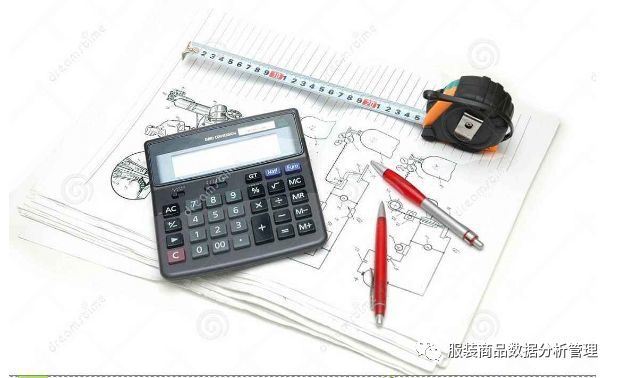# 店铺合理库存量的分析计算

X1=A+B+C+D+E+F+G+H+I+J+K+LX1=5╳16+3╳22+1╳20+28X4+1╳64+1╳32+1╳8+1╳20+1X12+4╳3+19╳20=806件，